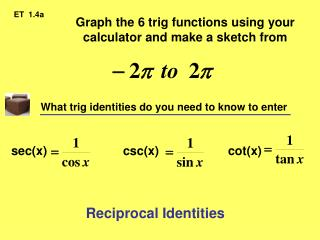# Graph the 6 trig functions using your calculator and make a sketch from - PowerPoint PPT PresentationDownload PresentationGraph the 6 trig functions using your calculator and make a sketch from

Graph the 6 trig functions using your calculator and make a sketch fromDownload Presentation## Graph the 6 trig functions using your calculator and make a sketch from

- - - - - - - - - - - - - - - - - - - - - - - - - - - E N D - - - - - - - - - - - - - - - - - - - - - - - - - - -
##### Presentation Transcript

1. ET 1.4a Graph the 6 trig functions using your calculator and make a sketch from What trig identities do you need to know to enter sec(x) csc(x) cot(x) Reciprocal Identities

2. y= cos x y= sin x y= sec x y= csc x y= cot x y= tan x

3. Find the limit (if it exists). If it does not, explain why. REMINDER OF DEF’N OF LIMIT (1.3 Notes – Don’t recopy) Book: DNE because decreases without bound Book: DNE because increases without bound Let f be a function and let a be a real number. exists iff Book: DNE because left & right limits don’t exist 1. Other answers: Left DNE Right DNE L not equal to R 2. 3.

4. Edwards’ definition of continuity: Don’t need to lift your pencil to draw the function. Causes of discontinuity: The road has been “hijacked” hole infinity jump (Removable Disc: canceling) Formal definition of continuity at x = a Note: Polynomials are always continuous.

5. a a a f(a) defined Limit Exists But f(a) not defined Limit Exists f(a) defined Limit at a DNE A function is continuous at a if: Yes Notice: The def’n removes of all of the above trouble spots. Are there other intervals on the graphs above that are continuous?

6. Greatest Integer Function: Check for Understanding Removable discontinuity? Continuous? NO Restated: Can you remove the discontinuity by simply redefining one point? NO Limit Exists Everywhere? NO

7. Check for Understanding. NO Continuous Everywhere? YES Continuous Over Domain? Removable discontinuity? NO Restated: Can you remove the discontinuity by simply redefining one point? NO Limit Exists Everywhere?

8. Check for Understanding. NO Continuous Everywhere? YES Continuous Over Domain? Removable Discontinuity? NO Restated: Can you remove the discontinuity by simply redefining one point? YES Plug the hole… Redefine the function using piece wise. Limit Exists Everywhere? How could you fix this discontinuity?

9. Check for Understanding. NO Continuous Over Domain? Removable Continuity? YES Restated: Can you remove the discontinuity by simply redefining one point? Limit Exists Everywhere? YES

10. Discuss the continuity of the function. x = - 8 x = -2 #1, #3 #3 x = 0 x = 3 #2, #3 #1, #2, #3 Where is the function not continuous? Using the def’n of limit, why?

11. Find the x-values (if any) at which f is not continuous. Which of the discontinuities are removable? x = 0 x = - 1 x = 1 Discontinuous at: What happens at the other discontinuities? What makes it removable? Canceling Infinity: Asymptotes

12. Continuity on Open vs. Closed Interval.Trick is to look at the end points. Continuous on (a, b) Not Continuous on [a, b] Continuous on (a, b) Continuous on [a, b] If we close these holes then we will have continuity on the closed interval [a, b]

13. Limit Properties vs. Continuity Properties SIDE BY SIDE VERY SIMILAR “If you start with good stuff, you’ll end up with good stuff.” True about f(g(x)) too!

14. Continuous at every point in theirdomain. are always continuous. • POLYNOMIAL • 2. RATIONAL • 3. RADICAL • 4. TRIGONOMETRIC Continuous provided denominator Continuous as long as radicand > 0 Continuous as long as not located at an asymptote.

15. Assignment 1.4a 3, 5, 7, 17, 21, 27, 29, 47, 49, 63, 69, 77, 79, 95, 103

16. ET 1.4b: Given s(t)= -16t2+500 If a construction worker drops a wrench from a height of 500 feet, how fast will the wrench be falling at exactly 2 seconds? Let t = 2 Then s(2) = -16(2)2 + 500 = 436 Select (a, f(a)) a  t Calculate slope of secant to estimate slope of tangent. 500 s(t) s(a) Compare to how you solved 1.3: #103 pg 69 t a t 2 t 2 (2) 2 + 2 = -64 ft/sec t 2

17. GREATEST CONSEQUENCE OF CONTINUITY. Intermediate Value Theorem Simply put… If a stalk of corn was 1 foot tall on a given day and then measured some time in the future at 5 feet, then the intermediate value theorem guarantees that the corn stalk must’ve been 4ft tall at some point and time. Insert picture of corn stalk. Reason: The growth of the stalk is continuous.

18. The Intermediate Value Theorem often can be used to locate zeros of a function that is continuous on a closed interval. • Specifically, if f is continuous on [a, b] and f(a) and f(b) differ in sign, the Intermediate Value Theorem guarantees the existence of at least one zero of f in the closed interval [a, b] .

19. Given some function f that is continuous on the closed interval [0, 1] If f(0) = negative f(1) = positive Then there exists x = c such that f(c) = 0 Intermediate Value Theorem, which relies on continuity, allows us to use the bisection method to find the zeros.

20. Assignment 1.4b 22, 30, 50, 72, 76, 82, 87, 91, 92, 108